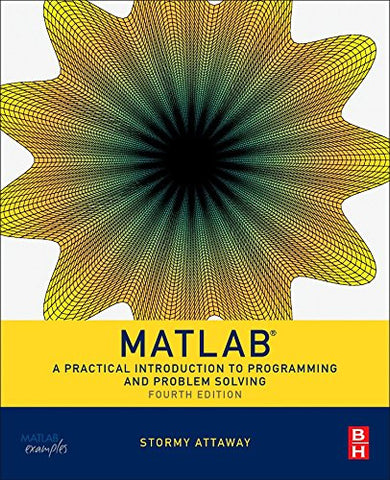Matlab, Fourth Edition: A Practical Introduction to Programming and Problem Solving

• ISBN-13: 9780128045251
• \$64.66

Only 6 left!

MATLAB: A Practical Introduction to Programming and Problem Solving, Fourth Edition, winner of a 2017 Textbook Excellence Award (Texty), has been updated to reflect the functionality of the current version of MATLAB, including the new H2 Graphics system. It features new and revised end-of-chapter exercises, more engineering applications to help the reader learn this software tool in context, and a new section on object-oriented programming in MATLAB. MATLAB has become the standard software tool for solving scientific and engineering problems due to its powerful built-in functions and its ability to program. Assuming no knowledge of programming, this book guides the reader both programming and built-in functions to easily exploit MATLAB's extensive capabilities for tackling engineering problems. The book starts with programming concepts, such as variables, assignments, and selection statements, moves on to loops, and then solves problems using both the programming concept and the power of MATLAB. In-depth coverage is given to input/output, a topic fundamental to many engineering applications.Winner of a 2017 Textbook Excellence Award (Texty) from the Textbook and Academic Authors AssociationPresents programming concepts and MATLAB built-in functions side-by-sideOffers a systematic, step-by-step approach, building on concepts throughout the book and facilitating easier learningIncludes sections on common pitfalls and programming guidelines to direct students toward best practicesCombines basic programming concepts, built-in functions, and advanced topics for problem solving with MATLAB to make this book uniquely suitable for a wide range of courses teaching or using MATLAB across the curriculum